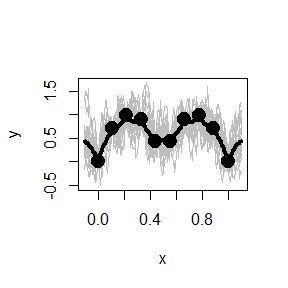# A Guide to the GauPro R package

#### 2017-09-10

This R package provides R code for fitting Gaussian process models to data. The code is created using the R6 class structure, which is why $ is used to access object methods. A Gaussian process fits a model to a dataset, which gives a function that gives a prediction for the mean at any point along with a variance of this prediction. Suppose we have the data below x <- seq(0,1,l=10) y <- abs(sin(2*pi*x))^.8 plot(x, y)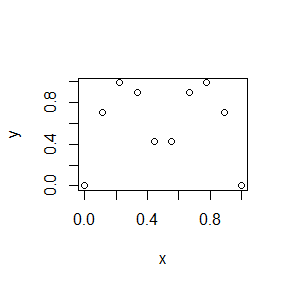A linear model (LM) will fit a straight line through the data and clearly does not describe the underlying function producing the data. lm_mod <- lm(y ~ x) plot(x, y) abline(a=lm_mod$coef, b=lm_mod$coef, col='red')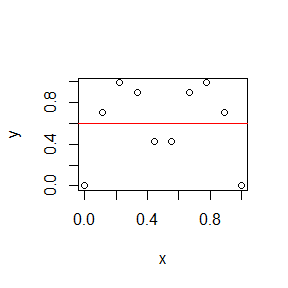A Gaussian process is a type of model that assumes that the distribution of points follows a multivariate distribution. In GauPro, we can fit a GP model with Gaussian correlation function using the function  library(GauPro) gp <- GauPro(x, y, parallel=FALSE) Now we can plot the predictions given by the model. Shown below, this model looks much better than a linear model. plot(x, y) curve(gp$predict(x), add=T, col=2)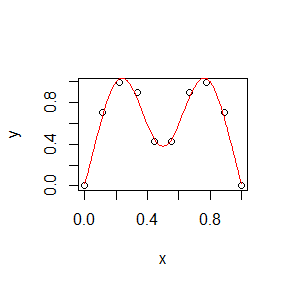A very useful property of GP’s is that they give a predicted error. The blue lines give an approximate 95% confidence interval. The width of the prediction interval is largest between points and goes to zero near data points, which is what we would hope for.

plot(x, y)
curve(gp$predict(x), add=T, col=2) curve(gp$predict(x)+2*gp$predict(x, se=T)$se, add=T, col=4)
curve(gp$predict(x)-2*gp$predict(x, se=T)$se, add=T, col=4)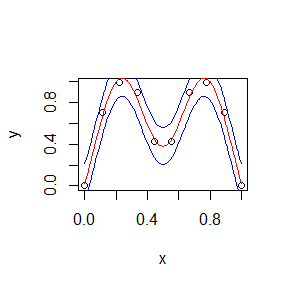GP models give distributions for the predictions. Realizations from these distributions give an idea of what the true function may look like. Calling plot on the 1-D gp object shows 20 realizations. There is no visible difference between the data points, only at the ends. if (requireNamespace("MASS", quietly = TRUE)) { plot(gp) }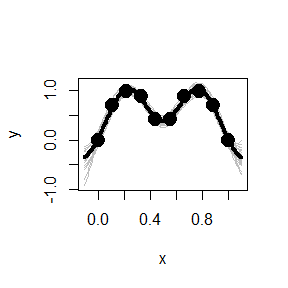## Using kernels The kernel, or covariance function, has a large effect on the Gaussian process being estimated. The function GauPro uses the squared exponential, or Gaussian, covariance function. The newer version of GauPro has a new function that will fit a model using whichever kernel is specified. To do this a kernel must be specified, then passed to GauPro_kernel_model$new. The example below shows what the Matern 5/2 kernel gives.

kern <- Matern52$new(0) gpk <- GauPro_kernel_model$new(matrix(x, ncol=1), y, kernel=kern, parallel=FALSE)
if (requireNamespace("MASS", quietly = TRUE)) {
plot(gpk)
}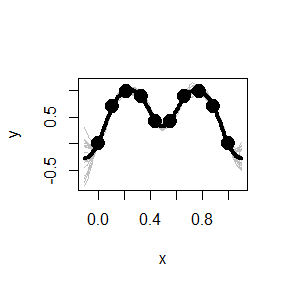The exponential kernel is shown below. You can see that it has a huge effect on the model fit. The exponential kernel assumes the correlation between points dies off very quickly, so there is much more uncertainty and variation in the predictions and sample paths.

kern.exp <- Exponential$new(0) gpk.exp <- GauPro_kernel_model$new(matrix(x, ncol=1), y, kernel=kern.exp, parallel=FALSE)
if (requireNamespace("MASS", quietly = TRUE)) {
plot(gpk.exp)
}